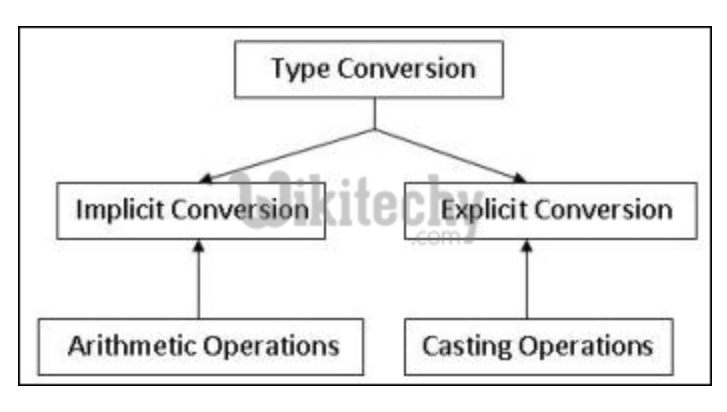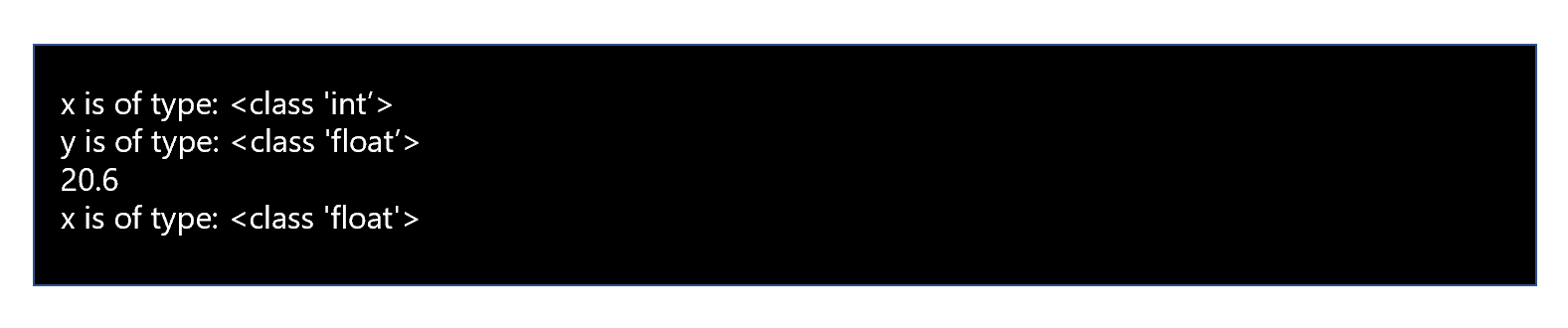# What is type conversion in python ?

• In python type casting functions to directly convert one data type to another which is useful in day-to-day and competitive programming.
• The types conversion consists of two types, they are implicit conversion and explicit conversion.

##Implicit Conversion

• In python implicit type conversion data types automatically converts one data type to another without any user involvement.

### Sample Code

``````x = 10

print("x is of type:",type(x))

y = 10.6

print("y is of type:",type(y))

x = x + y

print(x)

print("x is of type:",type(x))``````

### Output## Explicit Conversion

• In python explicit conversion data type is manually changed by the user as per their requirement.
• Int () function convertsany data type to integer. ‘Base’ specifies the base in which string is if the data type is a string.
• Float () function is used to convert any data type to a floating-point number.
• Ord () function is used to convert a character to integer.
• Hex () function is used to convert integer to hexadecimal string.
• Oct () function is used to convert integer to octal string.
• Tuple () function is used to convert to a tuple.
• Set () function returns the type after converting to a set.
• List () function is used to convert any data type to a list type.
• Dict () function is used to convert a tuple of order into a dictionary.
• Str () function is used to convert integer into a string.
• Complex () function converts real numbers to complex number.
• Chr () function converts number to its corresponding ASCII character.

### Sample Code

``````# Python code to demonstrate Type conversion

# using  tuple(), set(), list()

# initializing string

s = 'geeks'

# printing string converting to tuple

c = tuple(s)

print ("After converting string to tuple : ",end="")

print (c)

# printing string converting to set

c = set(s)

print ("After converting string to set : ",end="")

print (c)

# printing string converting to list

c = list(s)

print ("After converting string to list : ",end="")

print (c)``````

### Output## What is the Difference between C, C++, Java and Python ?

Answer : Developed Year,1972,1979,1991,1991

## What is Decorator in python ?

Answer : A decorator in Python is a function that takes another function as an argument…

## What is Double Asterisk (**) in python ?

Answer : In Python ** is an exponential operator……

## What is python unsigned right shift (>>>) ?

Answer : The symbol >>> is used to point out that what’s coming after is Python Code…..

## What is Python Wrapper ?

Answer : A wrapper is a python module that interface between python and another software library function which is a non python interface.

## What is Python Networking Programming ?

Answer : The standard library of python supports network protocols….